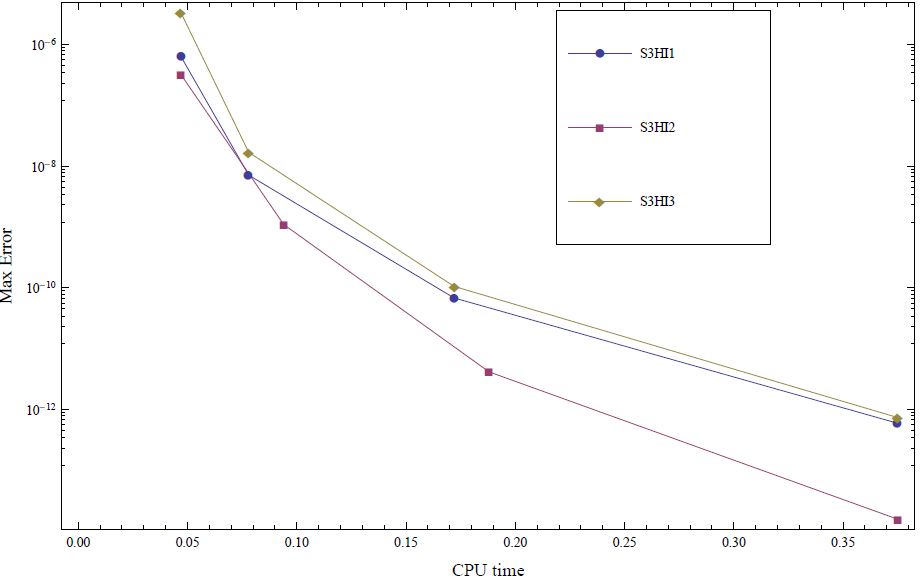# Computational study of a 3-step hybrid integrators for third order ordinary differential equations with shift of three off-step points

## Authors

• Mark I. Modebei Department of Mathematics Programme, National Mathematical Centre, Abuja, Nigeria
• Olumide O. Olaiya Department of Mathematics Programme, National Mathematical Centre, Abuja, Nigeria
• Ignatius P. Ngwongwo Department of Mathematics, Federal University of Technology, Owerri, Owerri, Nigeria

## Keywords:

Third Order Ordinary Differential Equation, Initial Value Problem, Boundary Value Problems, Linear Multistep, Hybrid Method

## Abstract

A Block of hybrid method with three off-step points based is presented in this work for direct approximation of solution of third-order Initial and Boundary Value Problems (IVPs and BVPs). This off-step points are formulated such that they exist only on a single step at a time. Hence, these points are shifted to three positions respectively in order to obtain three different integrators for computational analysis. These analysis includes; order of the methods, consistency, stability and convergence, global error, number of functions evaluation and CPU time. The superiority of these methods over existing methods is established numerically on different test problems in literature

Dimensions

J. Henderson & and K. R. Prasad, ”Existence and uniqueness of solutions of three-point boundary value problems on time scales”, Journal Nonlinear Science 8 (2001) 1.

C. P. Gupta & V. Lakshmikantham, ”Existence and uniqueness theorems for a third-order three point boundary value problem”, Journal of Nonlinear Analysis: Theory and Methods in Application 15 (1991) 949. DOI: https://doi.org/10.1016/0362-546X(91)90099-M

R. K. Sahi, S. N. Jator & N. A. Khan, ”Continuous Fourth Derivative Method For Third-order Boundary Value Problems”, International Journal of Pure and Applied Mathematics 85 (2013) 907. DOI: https://doi.org/10.12732/ijpam.v85i5.9

S. N. Jator, ”Novel Finite Di erence Schemes For Third Order Boundary Value Problems” International Journal of Pure and Applied Mathematics 53 (2009) 37.

O. Adeyeye & Z. Omar, ”Solving Third Order Ordinary Differential Equations Using One-Step Block Method with Four Equidistant Generalized Hybrid Points”, IAENG International Journal of Applied Mathematics (IJAM) 49 (2019) 1.

M. I. Modebei, O. O. Olaiya & A. C. Onyekonwu, ”A 3-step fourth derivatives method for numerical integrationof third order ordinary differential equations”, Int. J. Math. Ana Opt.; Theory and Applications 7 (2021) 32.

S. Islam, M. A. Khan, I. A. Tirmizi & E. H. Twizell, ”Non-polynomial splines approach to the solution of a system of third-order boundary-value problems”, Applied Mathematics and Computations 168 (2005) 152. DOI: https://doi.org/10.1016/j.amc.2004.08.044

P. K. Pandey, ”Solving third-order Boundary Value Problems with Quartic Splines”, SpringerPlus, 5 (2016) 1. DOI: https://doi.org/10.1186/s40064-016-1969-z

Y. Q. Hasan & S. A. Alaqel, ”Application of Adomian Decomposition Method to Solving Higher Order Singular Value Problems for Ordinary Differential Equations”, Asian Journal of Probability and Statistics 9 (2020) 28. DOI: https://doi.org/10.9734/ajpas/2020/v9i430232

A. Khan & P. Khandelwal, ”Numerical Solution of Third Order Singularly Perturbed Boundary Value Problems Using Exponential Quartic Spline”, Thai Journal of Mathematics 17 (2019) 663.

H. K. Mishra&S. Saini, ”Quartic B-Spline Method for Solving a Singular Singularly Perturbed Third-Order Boundary Value Problems”, American Journal of Numerical Analysis 3 (2015) 18.

Ra’ft Abdelrahim, ”Numerical solution of third order boundary value problems using one-step hybrid block method”, Ain Shams Engineering Journal 10 (2019) 179. DOI: https://doi.org/10.1016/j.asej.2018.02.003

B. S. H. Kashkari & S. Alqarni, ”Optimization of two-step block method with three hybrid points for solving third order initial value problems”, Journal of Nonlinear Science and Application 12 (2019) 450. DOI: https://doi.org/10.22436/jnsa.012.07.04

J. D. Lambert, ”Computational Methods in Ordinary Di erential Equations”, John Wiley, New York (1973).

M. I. Modebei, R. B. Adeniyi, S. N. Jator & H. C. Ramos, ”A block hybrid integrator for numerically solving fourth-order Initial Value Problems”, Applied Mathematics and Computation 346 (2019) 680. DOI: https://doi.org/10.1016/j.amc.2018.10.080

P. Henrici, ”Discrete Variable Methods in Ordinary Di erential Equations”, John Wiley & Sons, New York, USA, (1962).2021-11-29

## How to Cite

Mark I. Modebei, Olumide O. Olaiya, & Ignatius P. Ngwongwo. (2021). Computational study of a 3-step hybrid integrators for third order ordinary differential equations with shift of three off-step points. Journal of the Nigerian Society of Physical Sciences, 3(4), 459–468. https://doi.org/10.46481/jnsps.2021.323

## Section

Original Research